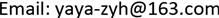BIPHYBiophysics2330-1686Scientific Research Publishing10.12677/BIPHY.2018.63005BIPHY-26562BIPHY20180300000_98678768.pdf数学与物理 生命科学 双层神经元网络中子网络的时空斑图研究 Spatio-Temporal Pattern in a Subnetwork of a Bi-Layer Neuronal Network 艳红1*福建师范大学，数学与信息学院&amp;福建省分析数学及应用重点实验室，福建 福州* E-mail:yaya-zyh@163.com2308201806035056© Copyright 2014 by authors and Scientific Research Publishing Inc. 2014This work is licensed under the Creative Commons Attribution International License (CC BY). http://creativecommons.org/licenses/by/4.0/Copyright © 2018 by author and Hans Publishers Inc.1. 引言

2. 噪声环境下的环–格双层耦合网络模型

Morris-Lecar神经元模型是描述北极鹅肌肉纤维的电活动的一个神经元模型。这个模型能模拟现实神经元的激发和恢复的特性。模型包含了一个产生快的动作电位的一个Ca2+流和一个K+流。与此同时，为了维持休止态处的恒定电位，还考虑了一个漏电流。二维的ML神经元模型由下面的微分方程给出：

{ C d V d t = − g L ( V − V L ) − g C a m ∞ ( V ) ( V − V C a ) − g K n ( V − V K ) + I , d n d t = λ n ( V ) ( n ∞ ( V ) − n ) , (1)

m ∞ ( V ) = 1 2 { 1 + tanh [ ( V − V 1 ) / V 2 ] } ,

n ∞ ( V ) = 1 2 { 1 + tanh [ ( V − V 3 ) / V 4 ] } ,

λ n ( V ) = λ ¯ n cosh [ ( V − V 3 ) / ( 2 V 4 ) ] ,

{ C d P i d t = − g C a 1 m ∞ ( P i ) ( P i − V C a ) − g K Q i ( P i − V K ) − g L ( P i − V L ) + I 1 + g 1 ( P i − 1 + P i + 1 − 2 P i ) + g 3 ( V i , i − P ) i + D ξ i , d Q i d t = λ n ( P i ) ( n ∞ ( P ) i − Q i ) , i = 1 , ⋯ , N . (2)

m ∞ ( P i ) = 1 2 { 1 + tanh [ ( P i − V 1 ) / V 2 ] } ,

n ∞ ( P i ) = 1 2 { 1 + tanh [ ( P i − V 3 ) / V 4 ] } ,

λ n ( P i ) = ϕ cosh [ ( P i − V 3 ) / ( 2 V 4 ) ] ,

{ C d V i , j d t = − g C a * m ∞ * ( V i , j ) ( V i , j − V C a ) − g K W i , j ( V i , j − V K ) − g L ( V i , j − V L ) + I 2 + g 2 ( V i − 1 , j + V i + 1 , j + V i , j − 1 + V i , j + 1 − 4 V i , j ) + g 3 ( P i − V i , i ) + D ξ i , j , d W i , j d t = λ n * ( V i , j ) ( n ∞ * ( V i , j ) − W i , j ) , i , j = 1 , ⋯ , N . (3)

m ∞ * ( V i , j ) = 1 2 { 1 + tanh [ ( V i , j − V 1 ) / V 2 ] } ,

n ∞ * ( V i , j ) = 1 2 { 1 + tanh [ ( V i , j − V 3 * ) / V 4 * ] } ,

λ n * ( V i , j ) = ϕ 2 cosh [ ( V i , j − V 3 * ) / ( 2 V 4 * ) ] ,

3. 环式子网络A的神经元放电行为和时空斑图

c i ( τ d ) = [ x i ( t ) − 〈 x i ( t ) 〉 ] [ x i ( t + τ d ) − 〈 x i ( t ) 〉 ] 〈 [ x i ( t ) − 〈 x i ( t ) 〉 ] 2 〉

4. 结论